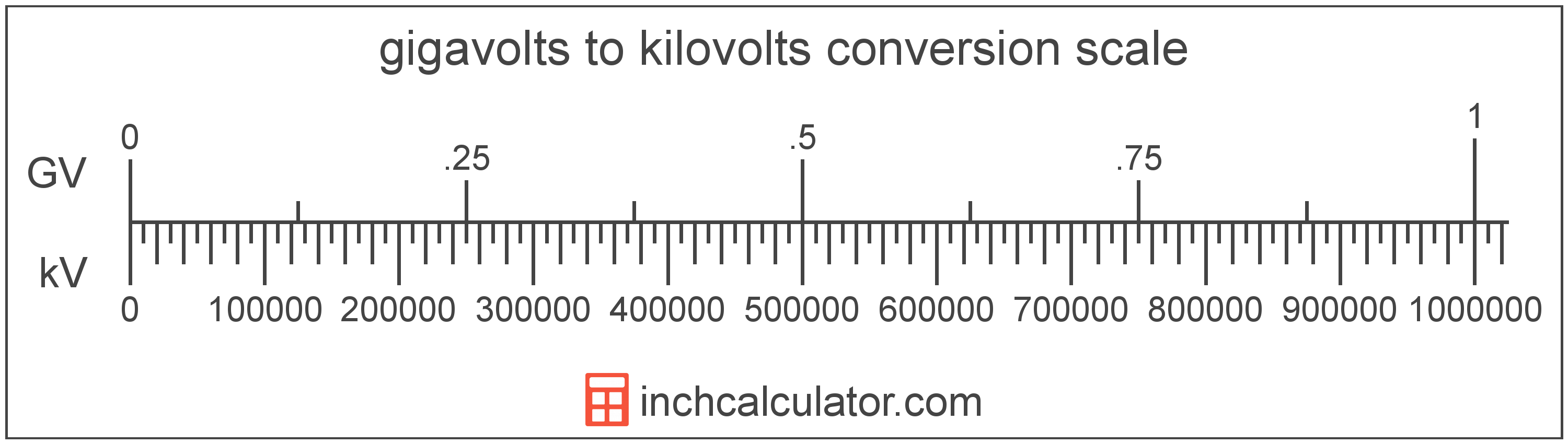# Gigavolts to Kilovolts Conversion

Enter the voltage in gigavolts below to get the value converted to kilovolts.

Results in Kilovolts:1 GV = 1,000,000 kV

## How to Convert Gigavolts to KilovoltsTo convert a gigavolt measurement to a kilovolt measurement, multiply the voltage by the conversion ratio.

Since one gigavolt is equal to 1,000,000 kilovolts, you can use this simple formula to convert:

kilovolts = gigavolts × 1,000,000

The voltage in kilovolts is equal to the gigavolts multiplied by 1,000,000.

For example, here's how to convert 5 gigavolts to kilovolts using the formula above.
5 GV = (5 × 1,000,000) = 5,000,000 kV

### How Many Kilovolts are in a Gigavolt?

There are 1,000,000 kilovolts in a gigavolt, which is why we use this value in the formula above.

1 GV = 1,000,000 kV

## Gigavolts

One gigavolt is equal to 1,000,000,000 volts, which are the potential difference that would move one ampere of current against one ohm of resistance.

The gigavolt is a multiple of the volt, which is the SI derived unit for voltage. In the metric system, "giga" is the prefix for 109. Gigavolts can be abbreviated as GV; for example, 1 gigavolt can be written as 1 GV.

## Kilovolts

One kilovolt is equal to 1,000 volts, which are the potential difference that would move one ampere of current against one ohm of resistance.

The kilovolt is a multiple of the volt, which is the SI derived unit for voltage. In the metric system, "kilo" is the prefix for 103. Kilovolts can be abbreviated as kV; for example, 1 kilovolt can be written as 1 kV.

## Gigavolt to Kilovolt Conversion Table

Gigavolt measurements converted to kilovolts
Gigavolts Kilovolts
0.000001 GV 1 kV
0.000002 GV 2 kV
0.000003 GV 3 kV
0.000004 GV 4 kV
0.000005 GV 5 kV
0.000006 GV 6 kV
0.000007 GV 7 kV
0.000008 GV 8 kV
0.000009 GV 9 kV
0.0000001 GV 0.1 kV
0.000001 GV 1 kV
0.00001 GV 10 kV
0.0001 GV 100 kV
0.001 GV 1,000 kV
0.01 GV 10,000 kV
0.1 GV 100,000 kV
1 GV 1,000,000 kV